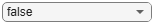Documentation

Train DDPG Agent to Swing Up and Balance Pendulum

This example shows how to train a deep deterministic policy gradient (DDPG) agent to swing up and balance a pendulum modeled in Simulink®.

For more information on DDPG agents, see Deep Deterministic Policy Gradient Agents. For an example that trains a DDPG agent in MATLAB®, see Train DDPG Agent to Control Double Integrator System.

Pendulum Swing-up Model

The reinforcement learning environment for this example is a simple frictionless pendulum that is initially hanging in a downward position. The training goal is to make the pendulum stand upright without falling over using minimal control effort.

Open the model.

mdl = 'rlSimplePendulumModel';
open_system(mdl)For this model:

• The upward balanced pendulum position is 0 radians, and the downward hanging position is pi radians.

• The torque action signal from the agent to the environment is from -2 to 2 Nm.

• The observations from the environment are the sine of the pendulum angle, the cosine of the pendulum angle, and the pendulum angle derivative.

• The reward ${\mathit{r}}_{\mathit{t}}$, provided at every time step, is:

${\mathit{r}}_{\mathit{t}}=-\left({{\theta }_{\mathit{t}}}^{2}+0.1{\stackrel{˙}{{\theta }_{\mathit{t}}}}^{2}+0.001{\mathit{u}}_{\mathit{t}-1}^{2}\right)$

where:

• ${\theta }_{\mathit{t}}$ is the angle of displacement from the upright position

• $\stackrel{˙}{{\theta }_{\mathit{t}}}$ is the derivative of the displacement angle

• ${\mathit{u}}_{\mathit{t}-1}$ is the control effort from the previous time step.

Create Environment Interface

Create a predefined environment interface for the pendulum.

env = rlPredefinedEnv('SimplePendulumModel-Continuous')
env =

Model: "rlSimplePendulumModel"
AgentBlock: "rlSimplePendulumModel/RL Agent"
ResetFcn: []
UseFastRestart: 'on'

The interface has a continuous action space where the agent can apply possible torque values from -2 to 2 Nm to the pendulum.

Set the observations of the environment to be the sine of the pendulum angle, the cosine of the pendulum angle, and the pendulum angle derivative.

numObs = 3;
set_param('rlSimplePendulumModel/create observations','ThetaObservationHandling','sincos');

To define the initial condition of the pendulum as hanging downward, specify an environment reset function using an anonymous function handle. This reset function sets the model workspace variable theta0 to pi.

env.ResetFcn = @(in)setVariable(in,'theta0',pi,'Workspace',mdl);

Specify the simulation time Tf and the agent sample time Ts in seconds

Ts = 0.05;
Tf = 20;

Fix the random generator seed for reproducibility.

rng(0)

Create DDPG agent

A DDPG agent approximates the long-term reward given observations and actions using a critic value function representation. To create the critic, first create a deep neural network with two inputs, the state and action, and one output. For more information on creating a deep neural network value function representation, see Create Policy and Value Function Representations.

statePath = [
imageInputLayer([numObs 1 1],'Normalization','none','Name','observation')
fullyConnectedLayer(400,'Name','CriticStateFC1')
reluLayer('Name', 'CriticRelu1')
fullyConnectedLayer(300,'Name','CriticStateFC2')];
actionPath = [
imageInputLayer([1 1 1],'Normalization','none','Name','action')
fullyConnectedLayer(300,'Name','CriticActionFC1','BiasLearnRateFactor',0)];
commonPath = [
reluLayer('Name','CriticCommonRelu')
fullyConnectedLayer(1,'Name','CriticOutput')];

criticNetwork = layerGraph();

View the critic network configuration.

figure
plot(criticNetwork)Specify options for the critic representation using rlRepresentationOptions.

Create the critic representation using the specified deep neural network and options. You must also specify the action and observation info for the critic, which you obtain from the environment interface. For more information, see rlRepresentation.

obsInfo = getObservationInfo(env);
actInfo = getActionInfo(env);
critic = rlRepresentation(criticNetwork,obsInfo,actInfo,'Observation',{'observation'},'Action',{'action'},criticOpts);

A DDPG agent decides which action to take given observations using an actor representation. To create the actor, first create a deep neural network with one input, the observation, and one output, the action.

Construct the actor similarly to the critic.

actorNetwork = [
imageInputLayer([numObs 1 1],'Normalization','none','Name','observation')
fullyConnectedLayer(400,'Name','ActorFC1')
reluLayer('Name','ActorRelu1')
fullyConnectedLayer(300,'Name','ActorFC2')
reluLayer('Name','ActorRelu2')
fullyConnectedLayer(1,'Name','ActorFC3')
tanhLayer('Name','ActorTanh')
scalingLayer('Name','ActorScaling','Scale',max(actInfo.UpperLimit))];

actor = rlRepresentation(actorNetwork,obsInfo,actInfo,'Observation',{'observation'},'Action',{'ActorScaling'},actorOpts);

To create the DDPG agent, first specify the DDPG agent options using rlDDPGAgentOptions.

agentOpts = rlDDPGAgentOptions(...
'SampleTime',Ts,...
'TargetSmoothFactor',1e-3,...
'ExperienceBufferLength',1e6,...
'DiscountFactor',0.99,...
'MiniBatchSize',128);
agentOpts.NoiseOptions.Variance = 0.6;
agentOpts.NoiseOptions.VarianceDecayRate = 1e-5;

Then, create the DDPG agent using the specified actor representation, critic representation and agent options. For more information, see rlDDPGAgent.

agent = rlDDPGAgent(actor,critic,agentOpts);

Train Agent

To train the agent, first specify the training options. For this example, use the following options:

• Run training for at most 50000 episodes, with each episode lasting at most 400 time steps.

• Display the training progress in the Episode Manager dialog box (set the Plots option) and disable the command line display (set the Verbose option).

• Stop training when the agent receives an average cumulative reward greater than -740 over five consecutive episodes. At this point, the agent can quickly balance the pendulum in the upright position using minimal control effort.

• Save a copy of the agent for each episode where the cumulative reward is greater than -740.

maxepisodes = 5000;
maxsteps = ceil(Tf/Ts);
trainOpts = rlTrainingOptions(...
'MaxEpisodes',maxepisodes,...
'MaxStepsPerEpisode',maxsteps,...
'ScoreAveragingWindowLength',5,...
'Verbose',false,...
'Plots','training-progress',...
'StopTrainingCriteria','AverageReward',...
'StopTrainingValue',-740,...
'SaveAgentCriteria','EpisodeReward',...
'SaveAgentValue',-740);

Train the agent using the train function. Training this agent is a computationally intensive process that takes several hours to complete. To save time while running this example, load a pretrained agent by setting doTraining to false. To train the agent yourself, set doTraining to true.

doTraining = false;
if doTraining
% Train the agent.
trainingStats = train(agent,env,trainOpts);
else
% Load pretrained agent for the example.
endSimulate DDPG Agent

To validate the performance of the trained agent, simulate it within the pendulum environment. For more information on agent simulation, see rlSimulationOptions and sim.

simOptions = rlSimulationOptions('MaxSteps',500);
experience = sim(env,agent,simOptions);